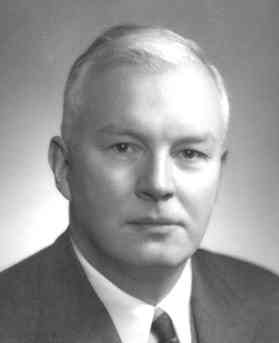Connecting...

# Currying and Partial Application by Marco Lopes

Have used the currying technique before?

Currying and partial application can often come up in your programming and knowing how to put it to use can be difficult. Scala Engineer, Marco Lopes helps us to have some clarity on these!

'In the previous installments we’ve mentioned currying and partial application. Let’s try to give some clarity as to what those two things mean.

#### Currying

Currying is a simple concept that is a bit hard to pass across, if you never came across it before. Let’s say you have a function 'f' that takes 3 'Int' values, 'x', 'y', and 'z' and returns a value 'r' of type 'String', in Scala the signature would look something like this 'def f(x: Int, y: Int, z: Int): String'. You can also think of it as a function that takes 'x' and returns another function that takes 'y' and 'z', and returns 'r', like 'def f(x: Int): (Int, Int) => String'. In turn, you can do the same thing with the return function and make it into a function that takes y, and returns a function that takes 'z' and returns 'r'. So, you end up with a function 'def f(x: Int): (Int => (Int => String))', or, because '=>' associates to the right, we can lose the parentheses, and end up with 'def f(x: Int): Int => Int => String', using syntax sugar 'def f(x:Int)(y: Int)(z: Int): String'.

What we did here, was we broke down our 3 parameter function into 3 functions that take 1 parameter each. The possibility of treating functions like this was developed and proved by mathematicians, amongst them Haskell Curry, after whom the technique was later named, as well as Haskell the language.#### Uses of currying

In Haskell, all functions are curried by default. This means that if you declare a function with more than one parameter, behind the scenes that functions just takes one parameter and returns another function that takes one parameter and so on. In Scala, functions are not inherently pure, so you have to manually curry a function, because if a function performs an effect the interpreter won’t know if it can partially apply it without breaking it’s procedural order, for example, in the case of a function that requests input from the user, that action doesn’t depend on the function inputs, but subsequent instructions on that function might. So, if you want a function to be curried, you have to explicitly say so and take responsibility to not break it. The example above would become 'def f(x:Int)(y: Int)(z: Int): String', meaning that the function is now curried.

But why would we want to curry a function? That takes us to the next section. Partial application.

#### Partial Application

If a function with arity greater than 1 (a function with more than 1 parameter), becomes a function with arity of 1 that returns another function, then, we can always call the function with only that parameter back. This is called partial application. So, for example, if you have the following function:You can always partially apply it, by calling the function without passing all of the three parameters, for example 'f 2' would return a function of type 'Int -> Int -> String' and 'f 2 3' would return a function of type 'Int -> String'. In Scala, the above example calls would be 'f(2)' and 'f(2)(3)', given that 'f' had been declared as curried.

An example of how this can be useful, can be seen with Haskell’s 'fmap' function, but not with Scala’s 'map' method due to Scala being OO and the thus the value we’re mapping over becoming an implicit instance on the method. So, the signature of 'fmap' is 'fmap :: Functor f => (a -> b) -> f a -> f b', so, we can do the following:The result would be 'Some 5', because we’re mapping the 'add2' function over the 'Some' value. Another thing we could do is partially apply 'fmap':Because ':t' shows the type of an expression, we will see that the type of 'add2InF' is 'add2InF :: Functor f => f Integer -> f Integer', we’ve partially applied 'fmap' to lift our 'add2' function so that it now can be applied to a value in the context of 'f' We can now do:which results in 'Just 7' *1

orWhich returns '[3,4,5]'

In Scala, currying and partial application are often used when a function require parameters that are configuration/dependencies and parameters that are the values it will act upon. That way, we can partially apply the configuration/common dependencies, and get back a function that takes only the values. For example:By passing a parser to 'transform', we get back a function that takes a value of 'A' and returns a value of 'B', which we can use with any 'A', without having to repeatedly pass the parser around.

It’s worth mentioning, that, as pschwarz commented bellow, in Scala you can curry functions at run time, so an adapted version of his example (with some minor changes for ease of understanding to newcomers):Next we’re going to take a look at type parameters, before diving into the basics of what is an effect system, and what benefits it can bring us.

*1This article was written by Marco Lopes and originally posted on marco-lopes.com